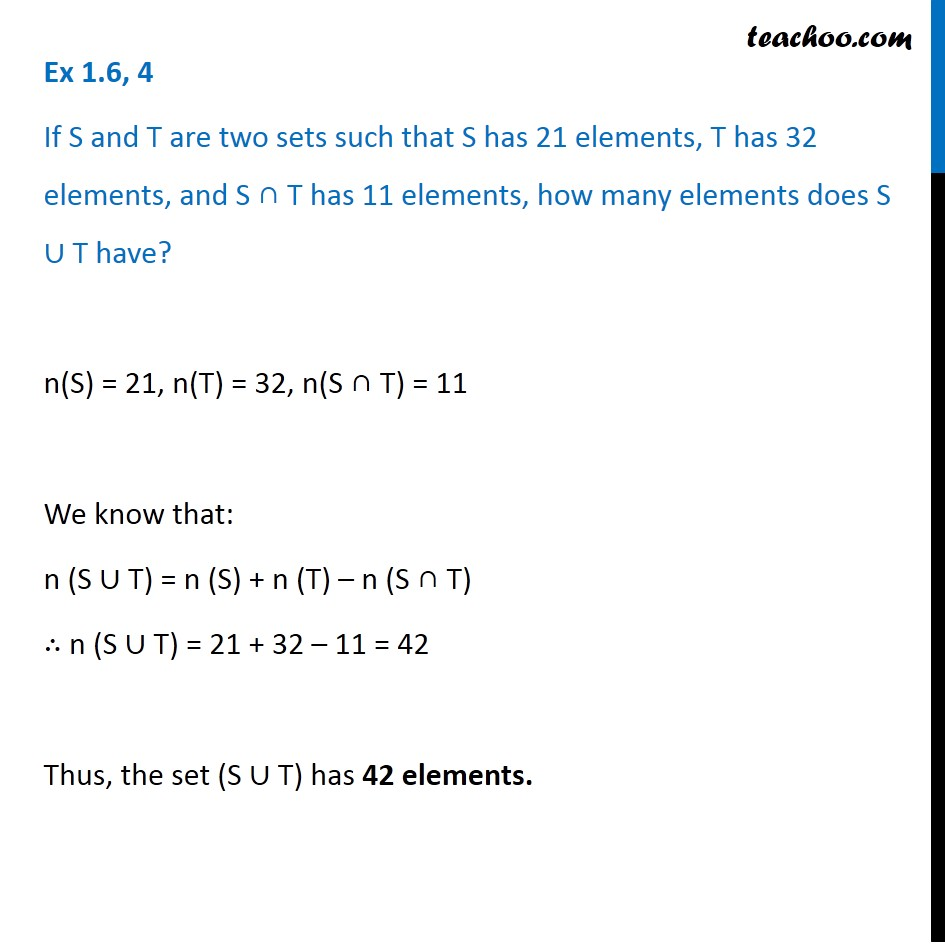Number of elements in Set Formula

Chapter 1 Class 11 Sets
Serial order wiseLearn in your speed, with individual attention - Teachoo Maths 1-on-1 Class

### Transcript

Question 4 If S and T are two sets such that S has 21 elements, T has 32 elements, and S ∩ T has 11 elements, how many elements does S ∪ T have? n(S) = 21, n(T) = 32, n(S ∩ T) = 11 We know that: n (S ∪ T) = n (S) + n (T) – n (S ∩ T) ∴ n (S ∪ T) = 21 + 32 – 11 = 42 Thus, the set (S ∪ T) has 42 elements.